# Propeller

The aircraft propeller rotates at an angular speed of 200 rad/s.

A) What is the speed at the tip of the propeller if its distance from the axis of rotation is 1.5 m?
B) What path does the aircraft travel during one revolution of the propeller at a speed of 540 km/h?

v =  300 m/s
s =  4.7124 m

### Step-by-step explanation: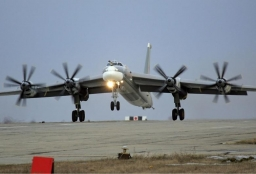Did you find an error or inaccuracy? Feel free to write us. Thank you!Tips to related online calculators
Do you want to convert velocity (speed) units?
Do you want to convert time units like minutes to seconds?

#### You need to know the following knowledge to solve this word math problem:

We encourage you to watch this tutorial video on this math problem:

## Related math problems and questions:

• Two aircraftFrom the airport will start simultaneously two planes, which fly tracks are perpendicular to each other. The first flying speed of 680 km/h and the second 840 km/h. Calculate how far the aircraft will fly for half an hour.
• Two aircraftTwo planes fly to the airport. At some point, the first airplane is away from the airport 98 km and the second 138 km. The first aircraft flies at an average speed of 420 km/h, the second average speed is 360 km/h, while the tracks of both planes are perp
• Constant Angular Acceleration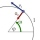The particle began to move from rest along a circle with a constant angular acceleration. After five cycles (n = 5), its angular velocity reached the value ω = 12 rad/s. Calculate the magnitude of the angular acceleration ε of this motion and the time int
• BomberThe aircraft flies at an altitude of 4100 m above the ground at speed 777 km/h. At what horizontal distance from the point B should be release any body from the aircraft body to fall into point B? (g = 9.81 m/s2)
• Circular motionThe mass point regularly moves in a circle with radius r = 3.4 m angular velocity ω = 3.6 rad/s. Calculate the period, frequency, and centripetal acceleration of this movement.
• Sound speed in Earth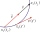Through the surface layers of the Earth, sound propagates 13 times faster than through air. During the geological survey, the charge exploded 1.7 km from the measuring station. How long does it take between the vibration of the soil at the measuring point
• The shooter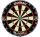The shooter heard the impact of the bullet on the target in one second after the shot. The bullet was moving at an average speed of 500 m/s. Calculate with speed of sound of 340 m/s. Determine the distance of the target.
• Two citiesCities A and B are 200 km away. At 7 o'clock from city A, the car started at an average speed of 80 km/h, and from B at 45 min later, the motorcycle is started at an average speed of 120 km/h. How long will they meet, and at what distance from point A wil
• Reconstruction of the corridorCalculate how many minutes will be reduced to travel 187 km long railway corridor, where the maximum speed increases from 120 km/h to 160 km/h. Calculate how many minutes will shorten travel time, if we consider that the train must stop at 6 stations, eac
• Two trains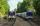Two trains go at each other. First at speed 40 km/h and the second at speed 60 km/h. At what distance will be 30 minutes before they will meet?
• Two airportsTwo airports are 2690 km away. From the first airport, the airplane flies at a speed of 600 km/h, from a second airplane at a speed of 780 km/h. When will they meet if they flew at 10:00? How far from the first airport?
• CarThe driver of a car is to get to 608 km distant city. From atlas found that 162 km will have to pass through the cities at an average speed 48 km/h. The remainder of the journey passes outside the cities at average speed 116 km/h. Calculate how many hours
• Cars 2Opava is 360 km distance from Prešov. At 8:00 hour, two cars started against each other from this cities at average speeds 88 km/h and 121 km/h. What hour will it meet and what distance it's travel if the car started from Prešov is faster?
• Cars motion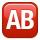From point A car start at the speed of 90 km/h. Against it departs from the point B at the same time another car at 60 km/h. For how long and where they met if the distance between A and B is 180 km?
• Aircraft bearingTwo aircraft will depart from the airport simultaneously, the first with a course of 30° and the second with a course of 86°. Both fly at 330 km/h. How far apart will they be in 45 minutes of flight?
• Two friendsTwo friends on a bicycle rode against each other from two places 49 km apart. The first set off at 8.00 at a speed of 20km/h, the second 12 minutes later at a speed of 25km/h. What time do they meet? How many km will each of them travel since then?
• CollisionThe two bodies, whose initial distance is 240 m, move evenly against each other consistently. The first body has an initial velocity of 4 m/s and an acceleration of 3 m/s2, the second body has an initial speed of 6 m/s and an acceleration of 2 m/s2. Fin